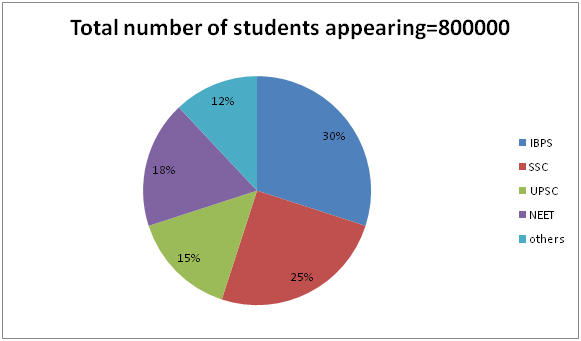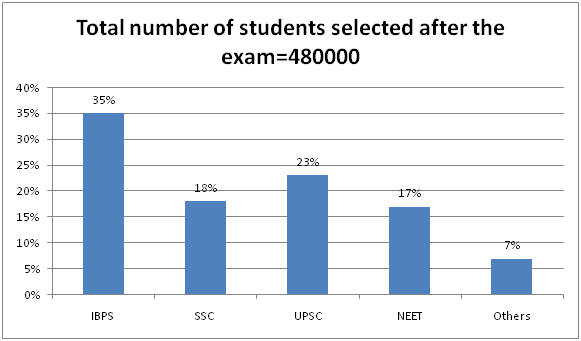# LIC HFL Assistant Manager Quantitative Aptitude Questions – (Day-05)

Dear Aspirants, Our IBPS Guide team is providing new series of Quantitative Aptitude Questions for LIC HFL Assistant Manager 2019 so the aspirants can practice it on a daily basis. These questions are framed by our skilled experts after understanding your needs thoroughly. Aspirants can practice these new series questions daily to familiarize with the exact exam pattern and make your preparation effective.

Check here for LIC HFL Mock Test 2019

Check here for IBPS RRB PO Mains Mock Test 2019

Check here for IBPS RRB Clerk Mains Mock Test 2019

### Click Here to Subscribe Crack High Level Puzzles & Seating Arrangement Questions PDF 2019 Plan

[WpProQuiz 7060]

1) A, B and C are deposited their amount in the bank with the respective ratio of 2:6:3. The total amount of all of them is Rs.5060. C invests the same amount to one of the scheme which is offering simple interest at the rate of x% for four years. After four years C received the interest amount which is average of the amount deposited by A and B. Then find the approximate value of x.

a) 22%

b) 33%

c) 44%

d) 55%

e) None of these

2) The present ages of A and B are in the ratio of 4:7 and age of A is half of the age of C. After 8 years B’s age is equal to the present age of C, then what is the present age of B?

a) 60 years

b) 56 years

c) 64 years

d) Cannot be determined

e) None of these

3) If the side of the square is equal to the radius of the circle and the cost of fencing of square field at the rate of Rs.20 per cm is Rs.560, then what is the cost of flooring of circular field at the rate of Rs.15 per cm?

a) Rs.2310

b) Rs.2180

c) Rs.1970

d) Rs.1860

e) None of these

4) The Shopkeeper marks the article which is 23% more than the cost price. If he sold the article for Rs.6642,then what is the original price of the article?

a) Rs.6000

b) Rs.4800

c) Rs.4200

d) Rs.5400

e) None of these

5) A man can row the boat x km downstream and the same distance upstream in 12 hours. Also he can row the boat can row 4x km downstream in 10 hours. In what time, he can row the x km distance in upstream?

a) 9.5 hours

b) 8.5 hours

c) 7.5 hours

d) 6.5 hours

e) None of these

Direction (6 – 10): Study the following information carefully and answer the given questions.

In 2017, the pie chart shows competitive exam-wise breakup of the number of students who appeared in the exam and the bar graph shows competitive-wise breakup and the number of students selected.6) The number of students selected in IBPS and SSC exam together is approximately what percent of the number of students appearing in NEET and UPSC exam together?

a) 98%

b) 96%

c) 94%

d) 92%

e) 90%

7) For which competitive is the difference between the number of students selected and the number of students appearing in the examination the minimum?

a) UPSC

b) NEET

c) Others

d) SSC

e) NEET & Others

8) Number of female students appearing in the NEET exam which is equal to the number of students selected from the SSC exam. If the ratio of male students appearing in the NEET exam to the number of male students appearing in the UPSC exam is 3:5, and then find the difference between the number of students selected from other exam and the number of female students appeared in UPSC exam?

a) 7600

b) 9800

c) 7800

d) 9600

e) None of these

9) In 2018, the number of students appearing the SSC exam is increased by 30% and the number of students appearing the exam in IBPS is decreased by 20%. The number of male students appearing the SSC exam in 2018 is 80% of the total number of students appearing the IBPS exam in 2018. The number of female students appearing the IBPS exam is 80000 more than that of number of female students appearing the SSC exam in 2018. What is the ratio of the number of female students appearing the SSC exam to the number male students appearing in IBPS exam?

a) 122:25

b) 253:12

c) 133: 7

d) 23:12

e) None of these

10) What is the Difference between number of students selected from NEET, IBPS and Other exams together and the number of students appearing in SSC and Others exam together?

a) 12800

b) 14000

c) 15800

d) 17600

e) None of these

Direction (1-5) :

Amount deposited by A= (2/11)*5060=Rs 920

Amount deposited by B= (6/11)*5060=Rs 2760

Amount deposited by C= (3/11)*5060=Rs 1380

Then, 1840=1380*x*(4/100)

x=33%

According to the question,

A/B=4/7

7A=4B

4B/7=A …………….. (1)

Also, A=C/2 …………. (2)

And, B+8=C

Using (2),

B+8=2A

Now, from (1), B+8=2(4B/7)

B+8=8B/7

7B+56=8B

B=56 years

Perimeter of the square=4a=(560/20)=28 cm

Then, Side of the square=28/4=7cm

Thus, Area of the circle=22/7*7*7=154cm2

Hence, Cost of flooring=154*15=Rs 2310

Original price=(100/123)*6642=Rs 5400

Let B be the speed of the boat in still water and W be the speed of the stream.

According to the question,

[x/(B+W)]+[x/(B-W)]=12

4x/(B+W)=10

x/(B+W)=5/2

Then, x/(B-W)=12-5/2

x/(B-W)=19/2 = 9.5 hours

Direction (6-10) :

Required percentage=53*4800*100/33*8000

=96%

Difference in IBPS=30/100*800000-35/100*480000

=240000-168000=72000

Difference in SSC=25/100*800000-18/100*480000

=200000-86400=113600

Difference in UPSC=15/100*800000-23/100*480000

=120000-110400=9600

Difference in NEET=18/100*800000-17/100*480000

=144000-81600=62400

Difference in Others=12/100*800000-7/100*480000

=96000-33600=62400

Number of female students appearing the NEET exam=18/100*480000=86400

Number male students appearing in NEET exam=(18/100*800000)-86400

=57600

Number of male students appearing in UPSC exam=5*57600/3

=96000

Number of female students appearing in UPSC exam=(15/100*800000)-96000

=24000

Number of students selected from other exam=7/100*480000=33600

Difference=33600-24000=9600

Number of students appearing in SSC exam in 2018=200000*130/100=260000

Number of students appearing in IBPS exam in 2018=240000*80/100=192000

Number of male students in SSC exam=192000*80/100=153600

Number of female students in SSC exam=260000-153600=106400

Number of female students in IBPS exam=106400+80000=186400

Number male students in IBPS exam=192000-186400=5600

Ratio=106400:5600=133: 7

Total number of students selected from NEET, IBPS, Other

=59/100*480000=283200

Total number of students appearing in SSC and Others

=37/100*800000=296000

Difference=296000-283200=12800